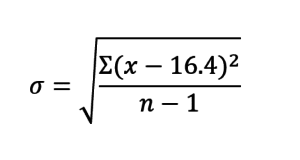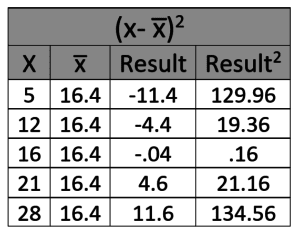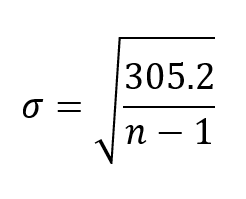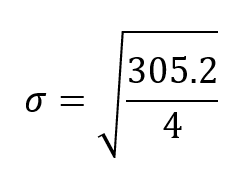# What is Standard Deviation?Standard deviation is a measure of variance within a data set.

It can be thought of as the average distance from the mean (calculated average) for each individual data point in a data set.

Standard deviation is a statistical operation that has wide applications, but for our purposes, we’re discussing it as it relates to the Six Sigma program.

As a reference, below is the standard deviation formula, along with a key and the appropriate order of operations.

## The Standard Deviation Formula## Key

Where:

σ = Lower case sigma is the symbol for standard deviation

Σ = Upper case sigma is the summation symbol

X = Each individual value in the data set

x̅ = The arithmetic mean (known as “x-bar”)

n = The number of data points in the set (the number of X values)

## Step 1

First, we will need to find the arithmetic mean of the data set so that we have a value for x-bar within the equation. This is the basic average formula: find the sum of all the numbers within the data set, then divide by the number of numbers.

Here we are describing doing things the old-fashioned way, but any spreadsheet software can find the average of a data set with the click of a button. In many cases, standard deviation is calculated as well. Therefore, though this formula is important, it’s often unnecessary to keep it in your memory.

## Step 2

Now we need to perform the (x - x̅)2 function.

On a computer, this is easy. With drag and drop formulas and copy and paste functions there is no need to write everything out.

If, however, you want to complete this calculation by hand, the best way to do it is to build a table like the one below. This demonstration table is constructed using the sample data set 5, 12, 16, 21, 28.

The average for this data set is 16.4.

Plugged into our equation, it now looks like this:And the results:Note that we are squaring each of the values. Later, we will be finding the square root of the result, but squaring numbers now means that there are no negative values. Negative values get messy when they are in the numerator or denominator of an equation.

## Step 3

Now that we have our results for x minus x-bar quantity squared, we see the uppercase sigma statistical operator in the equation. Simply add the values in the last column.

129.96 + 19.36 + .16 + 21.16 + 134.56 = 305.2

Plugged into our equation…There are five data points in the set, which means our n value is 5. Plug that into the equation and we get:Or, the square root of 305.2/4, which is 76.3.

The square root of that number rounds to 8.73, meaning that our formula is solved as

σ = 8.73

To put that another way, the average distance that any one data point is away from the average of 16.4 is 8.73.

To learn more about how sigma values are used in the world of Six Sigma, see our breakdown of the topic here, or check out this condensed version on Quora.

• Email
• More Networks
[class^="wpforms-"]
[class^="wpforms-"]
[class^="wpforms-"]
[class^="wpforms-"]
[class^="wpforms-"]
[class^="wpforms-"]
[class^="wpforms-"]
[class^="wpforms-"]
[class^="wpforms-"]
[class^="wpforms-"]
[class^="wpforms-"]
[class^="wpforms-"]
[class^="wpforms-"]
[class^="wpforms-"]
[class^="wpforms-"]
[class^="wpforms-"]
[class^="wpforms-"]
[class^="wpforms-"]
[class^="wpforms-"]
[class^="wpforms-"]
[523.251,1046.50]
[523.251,1046.50]
[523.251,1046.50]
[523.251,1046.50]
[523.251,1046.50]
[523.251,1046.50]
Share via